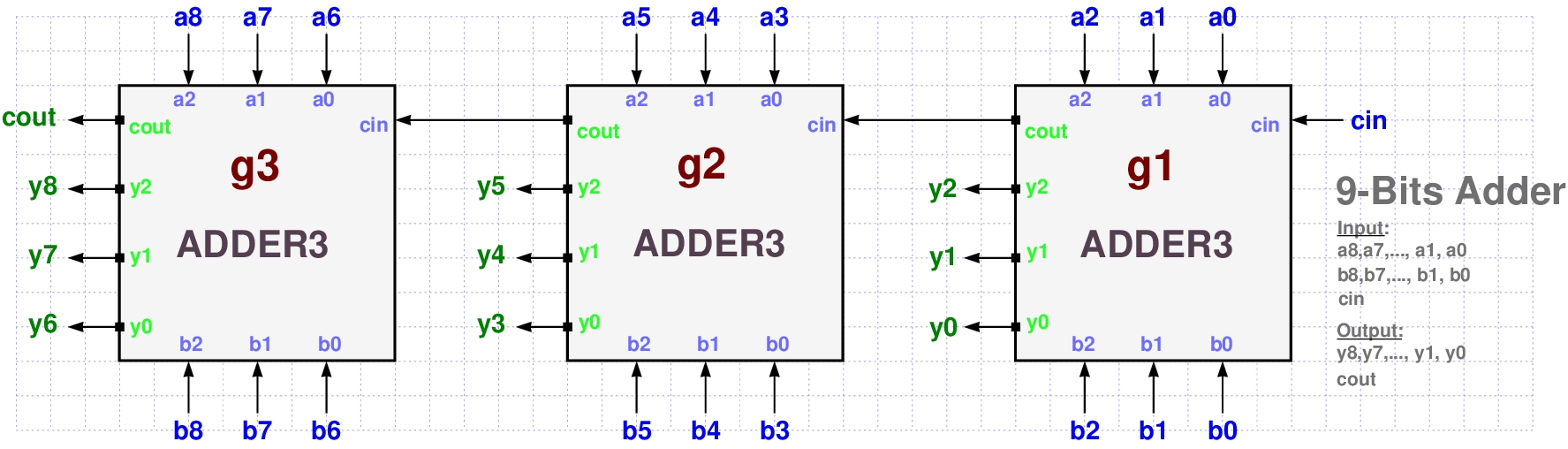# PyCircPL - Python Logic Circuit Programming Language

• The Google Colaboratory notebook has the advantage that you can run PyCircPL code from it without having to install PyCircPl or even Python on your local system.
• You first need to copy it to your google drive (or github repository)
• You can also dowload it to your local device and open it as a Jupyter notebook (if you have it installed with your Python).

## Installing the PyCircPL package¶

• The PyCircPL package can be installed on your local system by running the following command from the command line
pip install pycircpl
• Or you may try running one of the following commands from this notebook.
• If you are running it from a Jupyter notebook on your local system, then it will be installed on your device.
• After installation, you may need to restart this notebook.

• After installing the PyCircPL package, you need to import it.
• The following command imports PyCircPL to your Python interpreter.

## Introduction¶

• PyCircPL is a simple Python package for simulating Logic Circuits specifically designed for educational use in introductory computation and digital logic college courses.
• As such, it was primarily tuned for simplicity, readability, convenience, and fast learning curve.
• Less for speed or industrial production.
• It is a lightweight package especially designed for small to medium scale circuits, such as those that are studied in introductory academic courses on the theory of computation and electronic digital design.
• Its main characteristic is that a digital circuit or a boolean formula can be easily defined by a series of simple Python commands, rather than an external static language.
• So, the only requirement is basic knowledge of the Python programming language, with a little programming skill.
• Experienced Python programmers can probably benefit a lot more from this package.
• It can be a useful companion for theoretical courses on computation models and languages who wish also to engage the students with some programming experience and skills.
• It is planned to be used in such a course by the author (Hebrew book at http://samyzaf.com/afl.pdf).
• It enables students to easily model and experiment with
• Typical logic circuit design
• Logic Circuit or Boolean formula Validation
• Logic Circuit Testing
• Logic problem solving
• It does provide an opportunity for students to develop and practice some programming skills while covering the theoretical computation course.
• In this tutorial, we will cover:
1. Basic usage of PyCircPL for simulating Logic Circuits or Boolean formulas.
2. A short survey of the commands and tools of the PyCircPL package.
3. Advanced usage of PyCirc for experienced Python programmers (TODO).

## Example 1: Simulating the circuit FOO¶

• We start with a very simple logic circuit which we call "FOO" whose PyCirc Diagram is given below

• Inputs gates: $x_1$, $x_2$, $x_3$
• Output gates: $y_1$, $y_2$
• Logic formula: $(y_1, y_2) = (x_1 \land x_2 , \ \neg x_3)$
• See https://www.samyzaf.com/pycirc/pycirchdl.html for detailed information on PyCirc diagrams.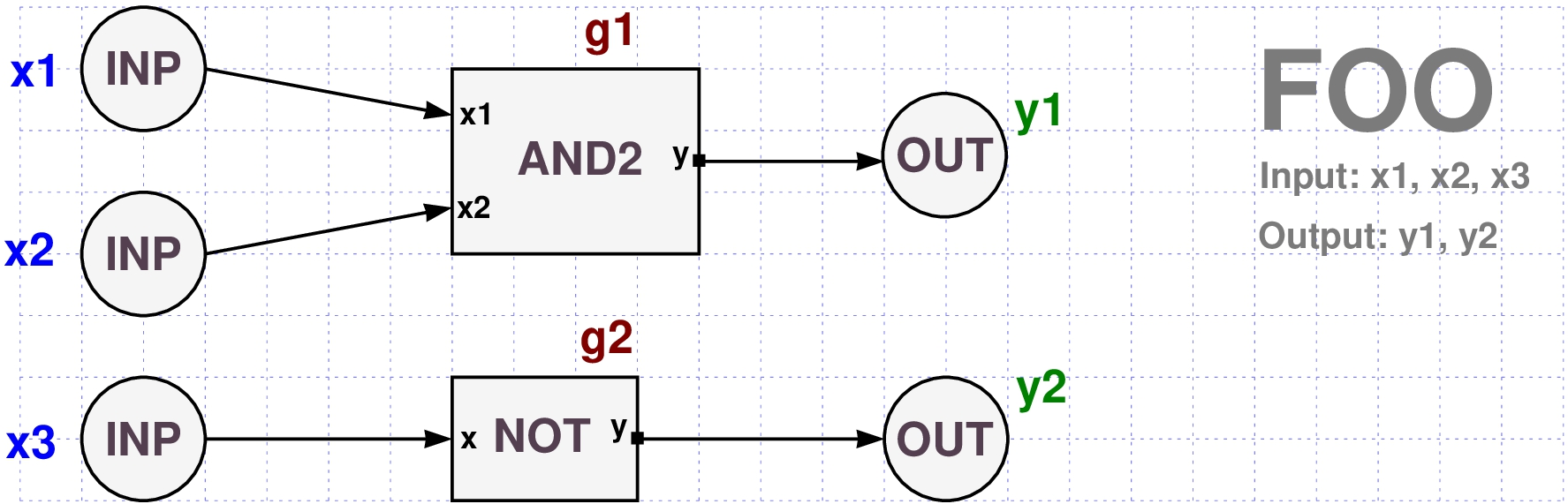• Here is the PyCircPL code for modeling this circuit:

• Notic that this is a pure Python code!

• So you need to run its cell in order to execute it.
• A circuit definition starts with the stabdard Python keyword def which is used to define a Python function.

• The function name is identical to the circuit name FOO.
• The function argument list is identical to the circuit boolean input list x1, x2, x3.
• The function output list (in the last return statement) is identical to the circuit output list.

• Note that we could make the definition shorter by removing the two intermediate variable g1 and g2, but their presence keeps a resemblance with the circuit diagram.

• We ephasize again: the above definition of FOO is a pure Python function!

• This means that you can use in any othe Python code and in other programs/scripts for building new circuit functions.

• Here is a simple example which computes the circuit for the input

x1 = 1
x2 = 1
x3 = 0


Here is a more sophisticated example for Pyhton programmers for generating the Truth Table of FOO. That is, all possible outputs.

• After successful design of a circuit function such as FOO, it can be placed in a single python code file and loaded as usual with the Python import command.
• You may want to copy the function library that we use in this notebook to your local pc.
• Here is a link to the Python file that contains all functions that we use in this notebook:

## Example 2: Simulating the circuit FRED¶

• Here is an example of cell called FRED which uses an instance of the cell FOO as one of its building blocks.
• Note that this cell is using a gate of type FOO whose function we defined in Example 1 above.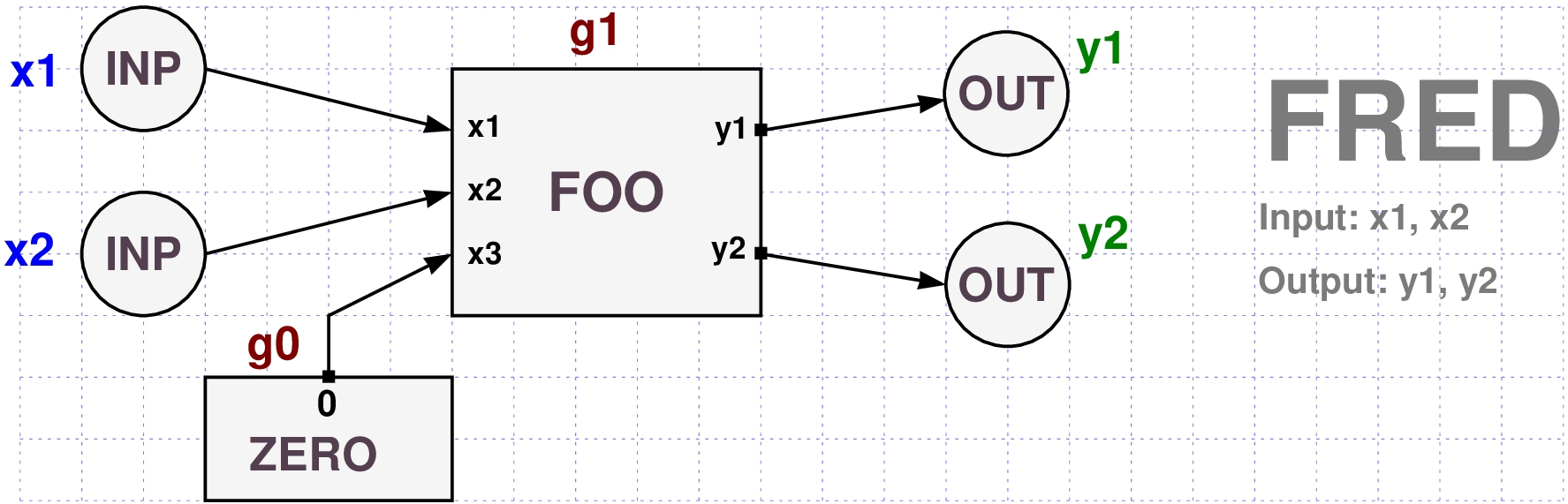• It is easy to write its corresponding function.
• Note that the function FOO is used in the function FRED.

## Example 3: The circuit HAM¶

• The follwing cell HAM contains two gates of type FOO and two gates of type XOR3.
• The XOR3 cell is a typical xor cell with 3 input gates.
• It also contains one gate of type NOT.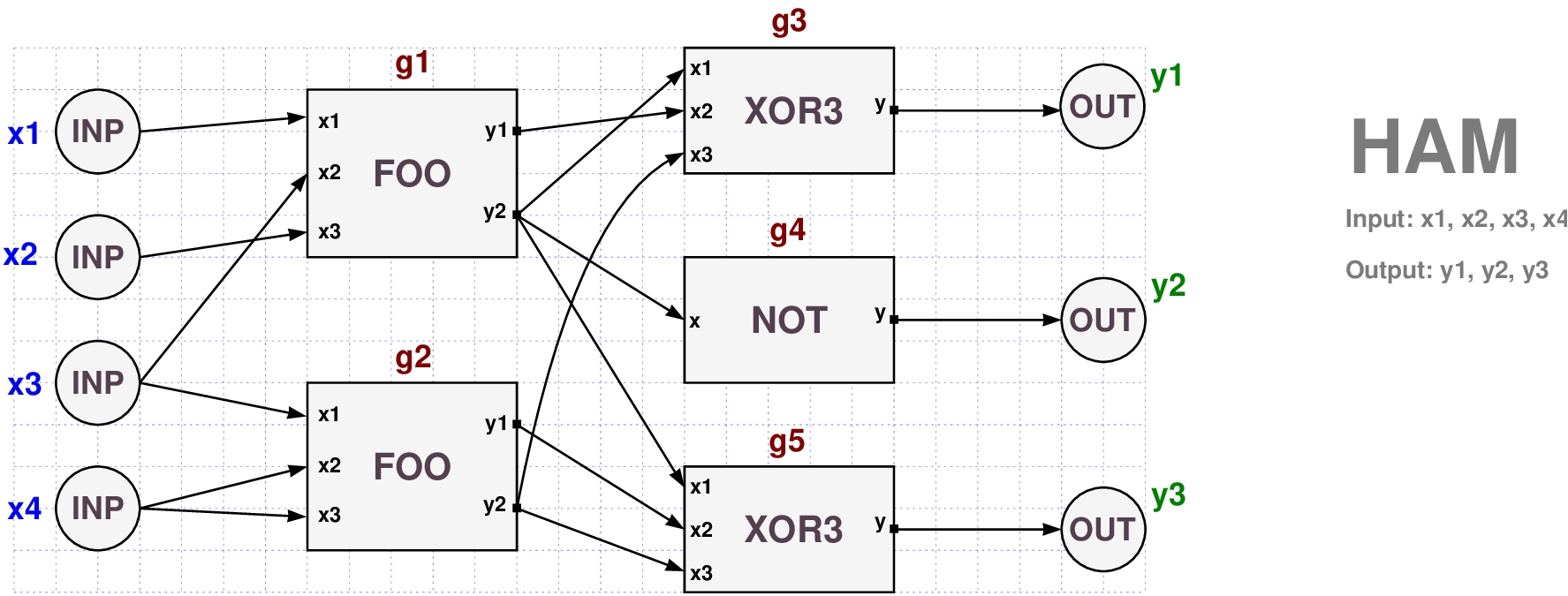• Here is a PyCircPL code for modeling this cell.
• Notice lines 2 and 3 of the code that use the function FOO.
• Here is a simple code for generating the truth table of HAM.
• We use the product utility from the itertools module (which is automatically loaded by pycircpl) to generate all the possible combinations of 4 boolean values.

## Example 4: Simulating 1-bits counter circuit¶

• The following circuit (given simple digraph represantation) counts the number of 1-bits of its input list.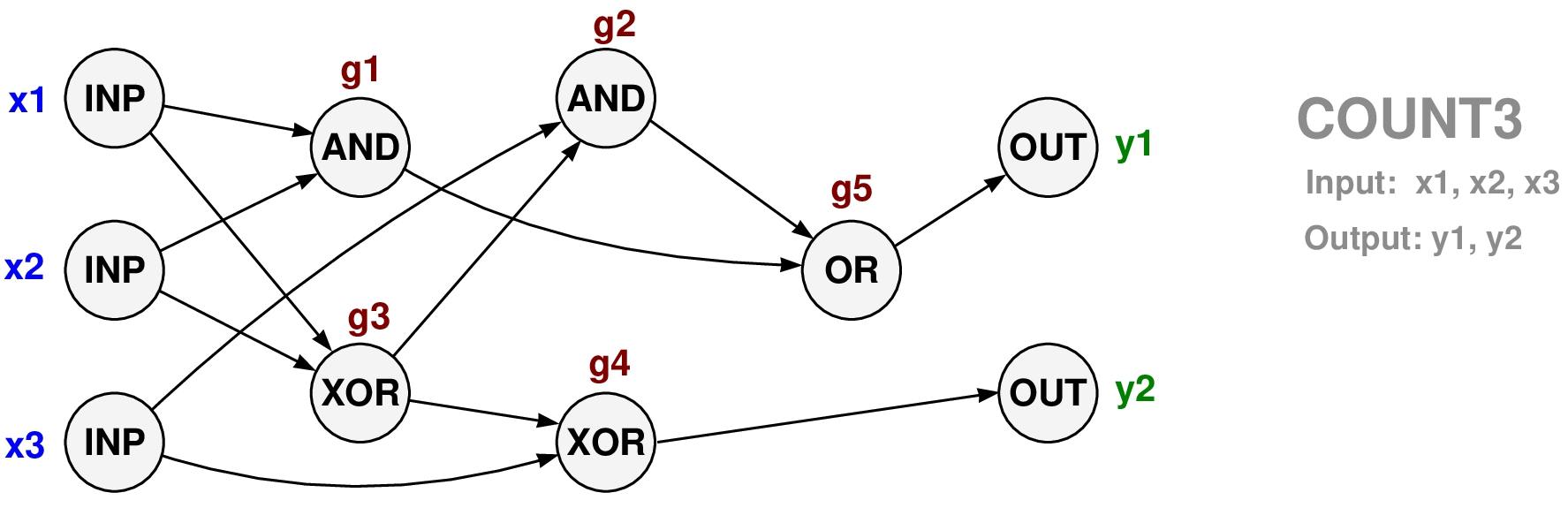• This is the correponging Python function:
• Converting the graph diagram to the corresponding Python code is an easy task.
• Each non-input gate g1, g3, g2, g4, g5, y1, y2, is converted to its corresponding Python statement by simply applying its logical operator on its inputs.
• Note that gate order is critical! You have to go from low to high depth.
• For example, gate variable g3 must be defined befor g2 since it is an input to g2!
• For example, here is what happens we apply COUNT3 on the input list
x1 = 1 ; x2=0 ; x3 = 1
• The number of 1-bits in the input (1,0,1) is 2 which in binary form is (1,0) as the above calculation shows.
• It is easy to verify all possible outcomes of this circuit function with the following code:
• Note that the same applies if we are given a boolean formula of this logical circuit $$\begin{array}{rcl} y_1 &=& (x_1 \wedge x_2) \vee ((x_1 \oplus x_2) \wedge x_3) \\ y_2 &=& (x_1 \oplus x_2) \oplus x_3 \end{array}$$ instead of its digraph representation.
• In most cases, the digraph diagram is more intuitive, and easier to work with than the algebraic formula.

## Example 5: Simulating a 4x1 Multiplexer¶

• Multiplxers are important circuit elements in electronic design.
• Here is a simple PyCirc Diagram for a 4x1 Multiplexer circuit (aka MUX2)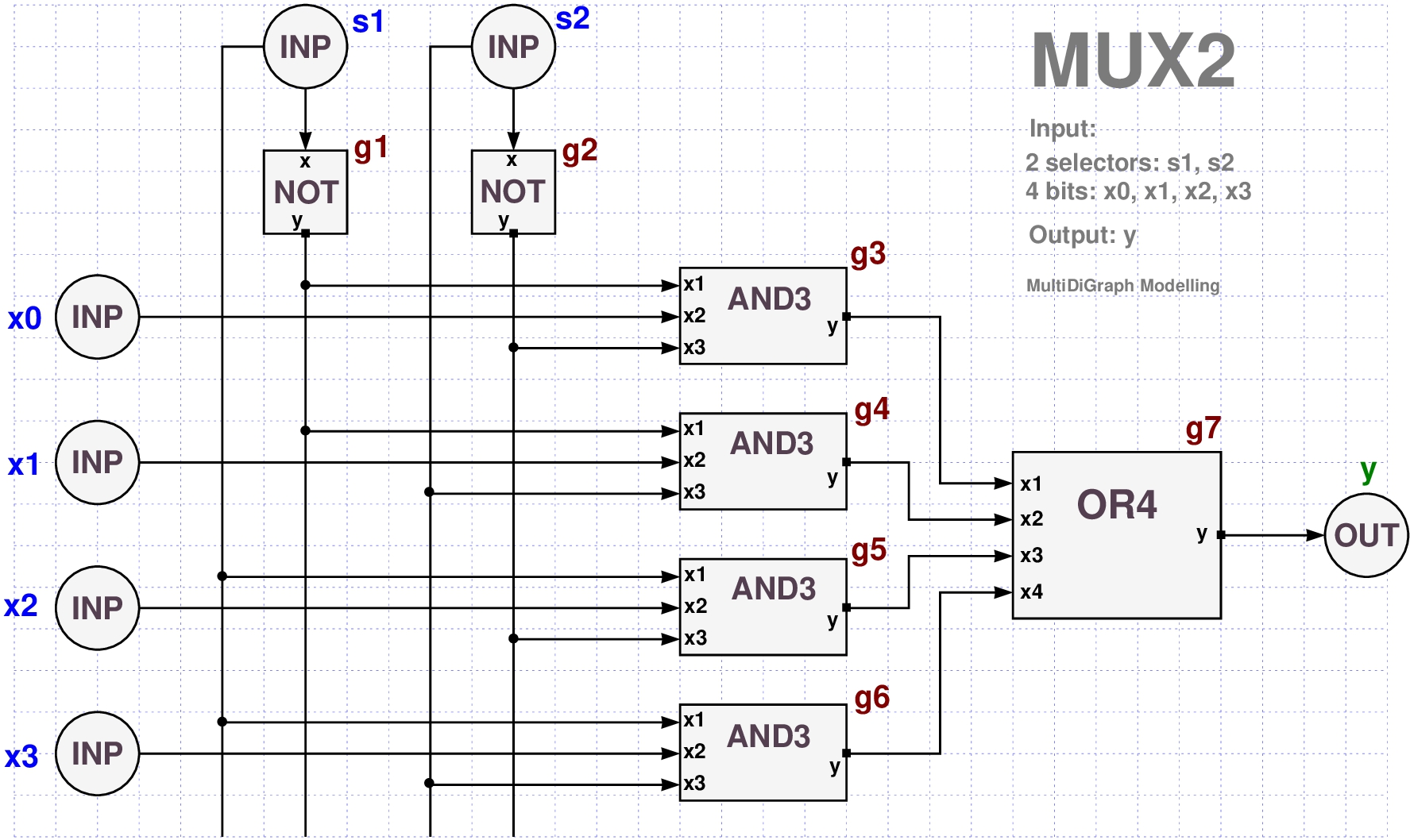## Example 6: Simulating a 8x1 Multiplexer circuit¶

• Now we build a function for a 8x1 Multiplexer circuit (aka MUX3)
• Note that the 8x1 Multiplexer diagram is using our MUX2 circuit as one of its building blocks (two instance of MUX2 are needed).
• We also need one instance of 2x1 Multiplexer (aka MUX1), which we leave to the student as an easy exercise.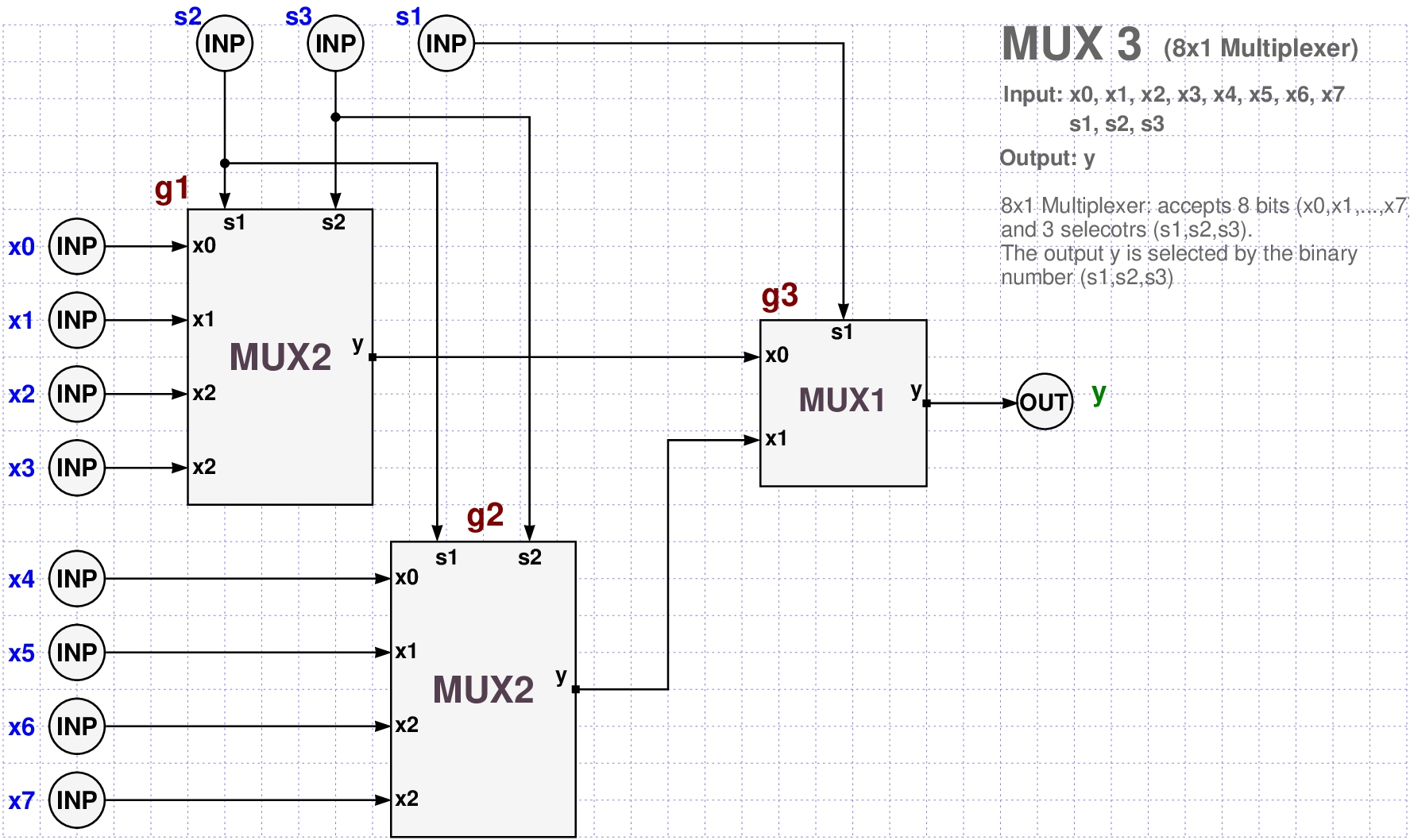• As you can see from the diagram we now have 8 inputs bits: x0, x1, x2, x3, x4, x5, x6, x7,
and one output bit: y.
• We only need 3 logic gates: g1, g2, and g3.
• g1 and g2 are two instances of MUX2,
• g3 is an instance of MUX1.
• Here is the code for creating a PyCircPL MUX3 function.
• We leave to the student to figure out the MUX1 function as an easy exercise.
• Using the already defined MUX1 and MUX2 functions, it took 5 lines to define the MUX3 function!
• Here is a simple call of MUX3 on a simple input.
• In the next example we sample 3% of the truth assignments to the MUX3 input and check the output.

• Here is a simple design for 3 bits adder with carry in (cin) and carry out (cout) bits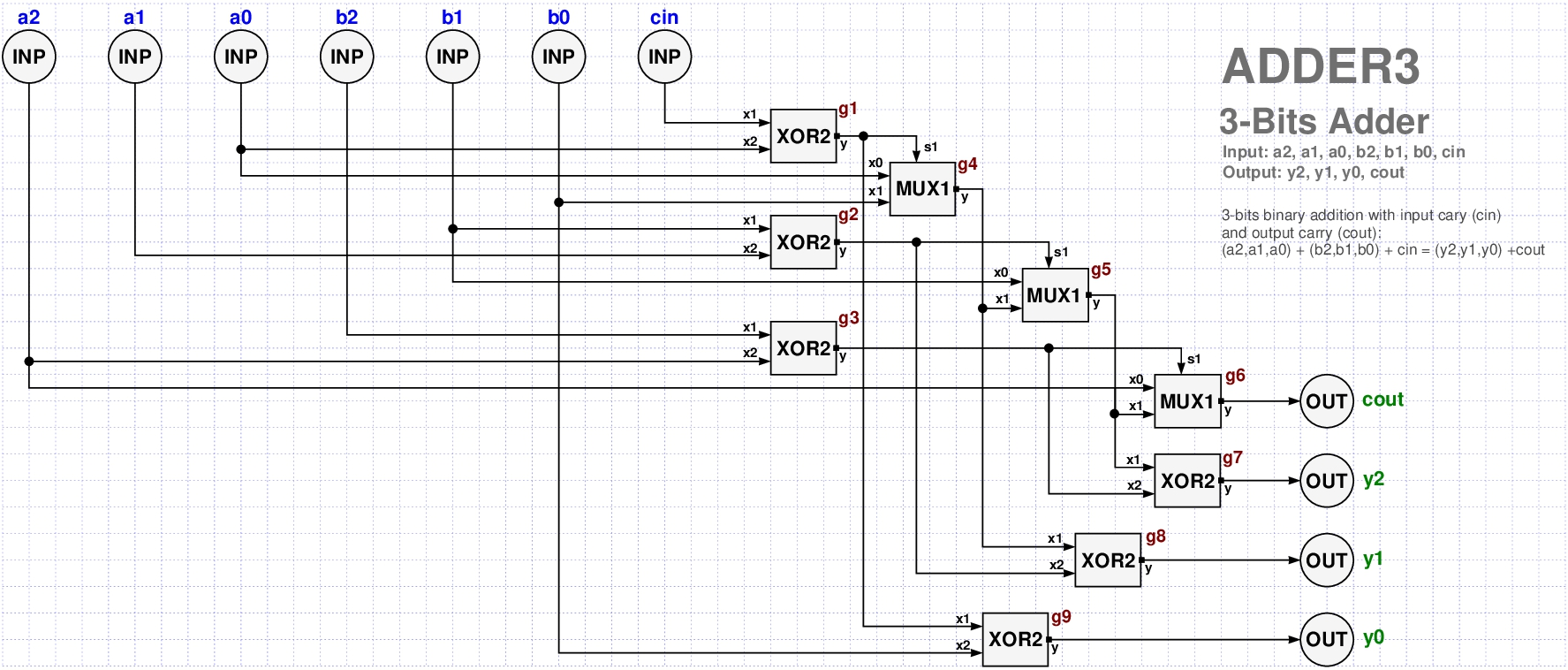• This circuit acceps three types of input
• two binary numbers: $(a_2,a_1,a_0)$, $(b_2,b_1,b_0)$
• a carry in bit: cin.
• Its output $(y_2,y_1,y_0)$ is the binary sum of the two numbers (with the carry added).
• In case of addition overflow, we need a carry out (cout) output bit as well.
• The following PyCircPL code describes a model for the ADDER3 cell.
• For example, lets try to compute 001+001

This result should be of course interpreted as follows

cout=0, y2=0, y1=1, y=0


which is the correct result 10.

• Input: a<8:0> + b<8:0> + cin
• Output: y<8:0> + cout